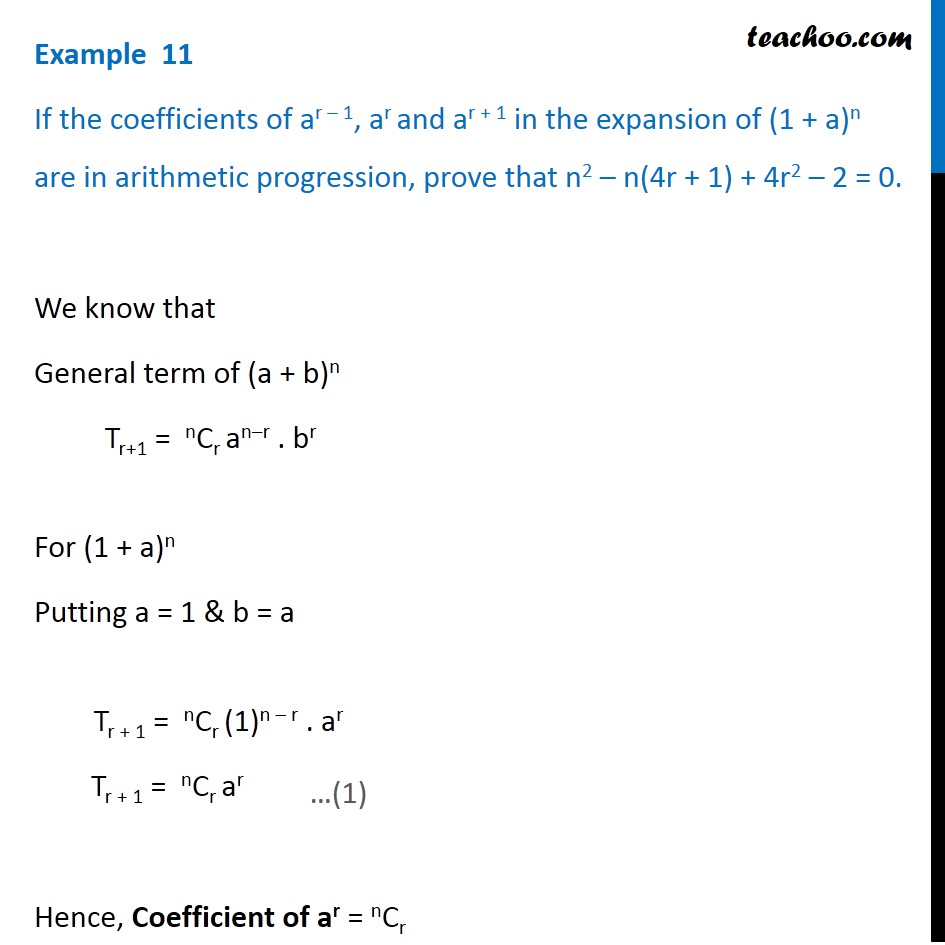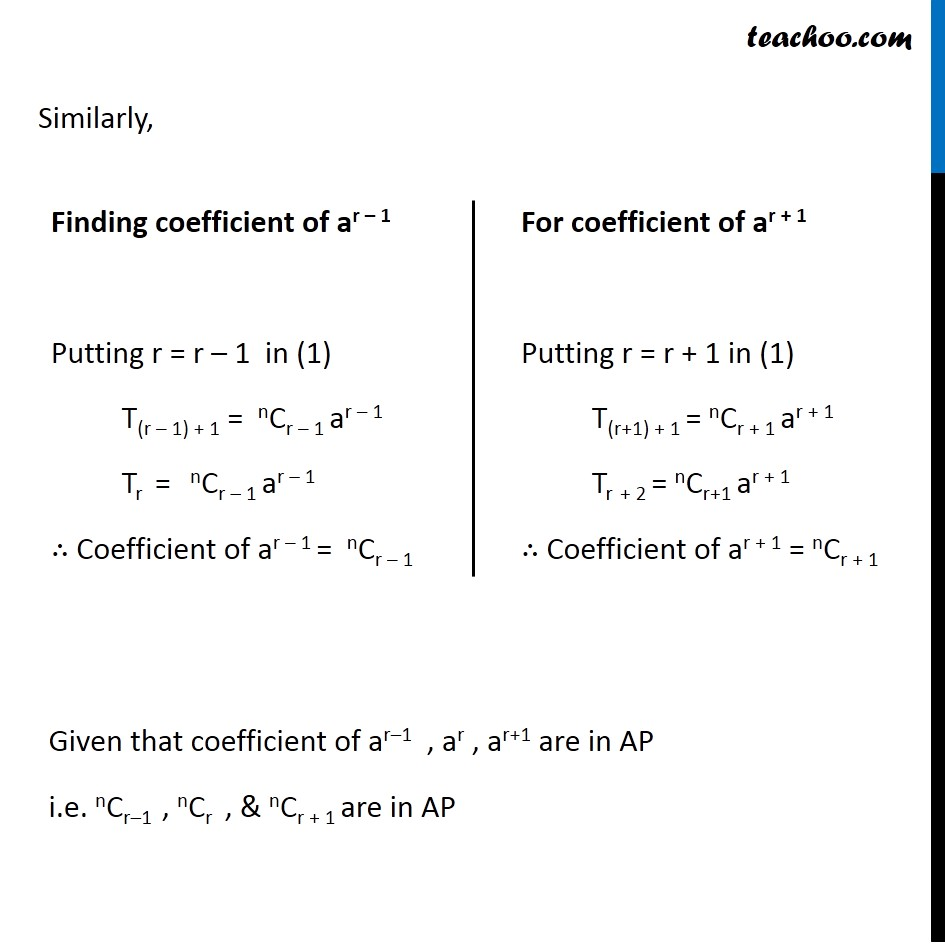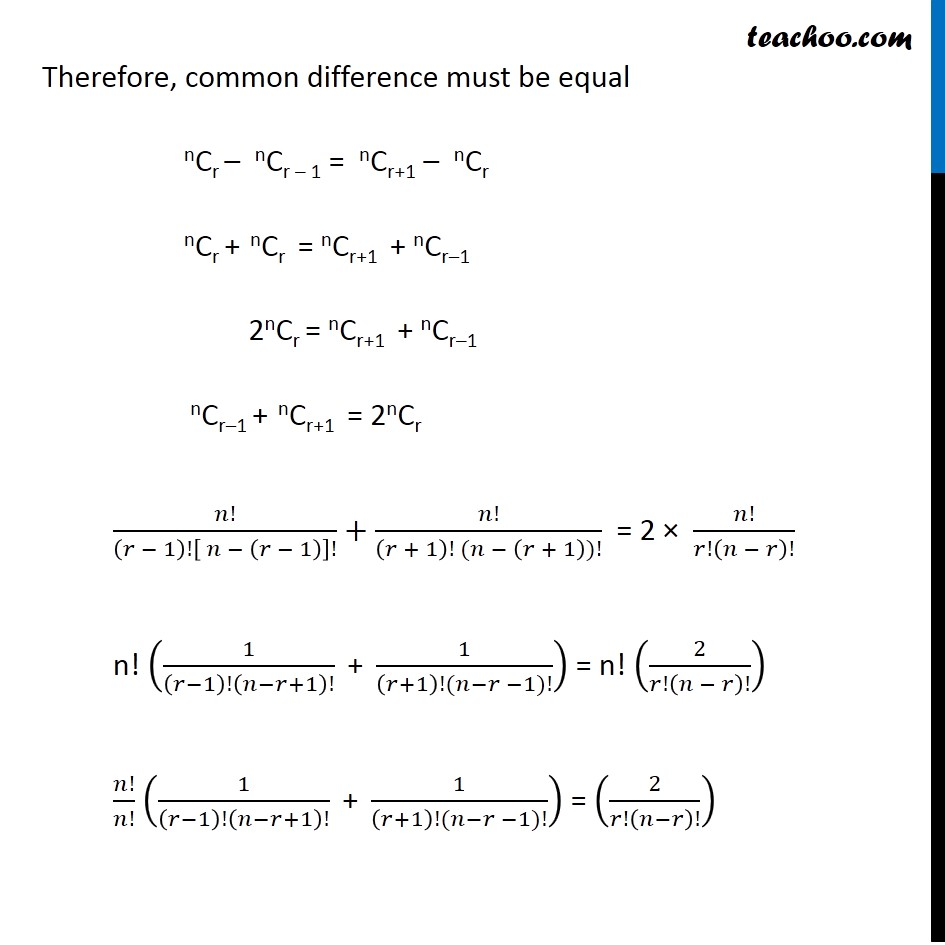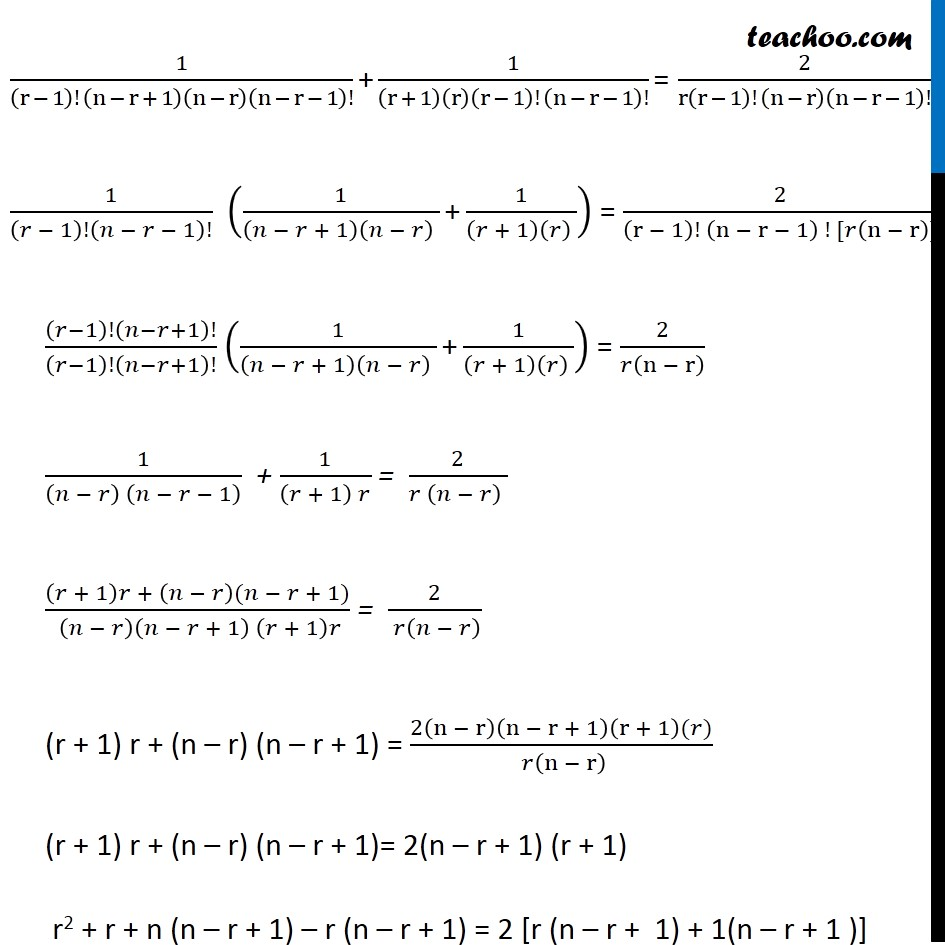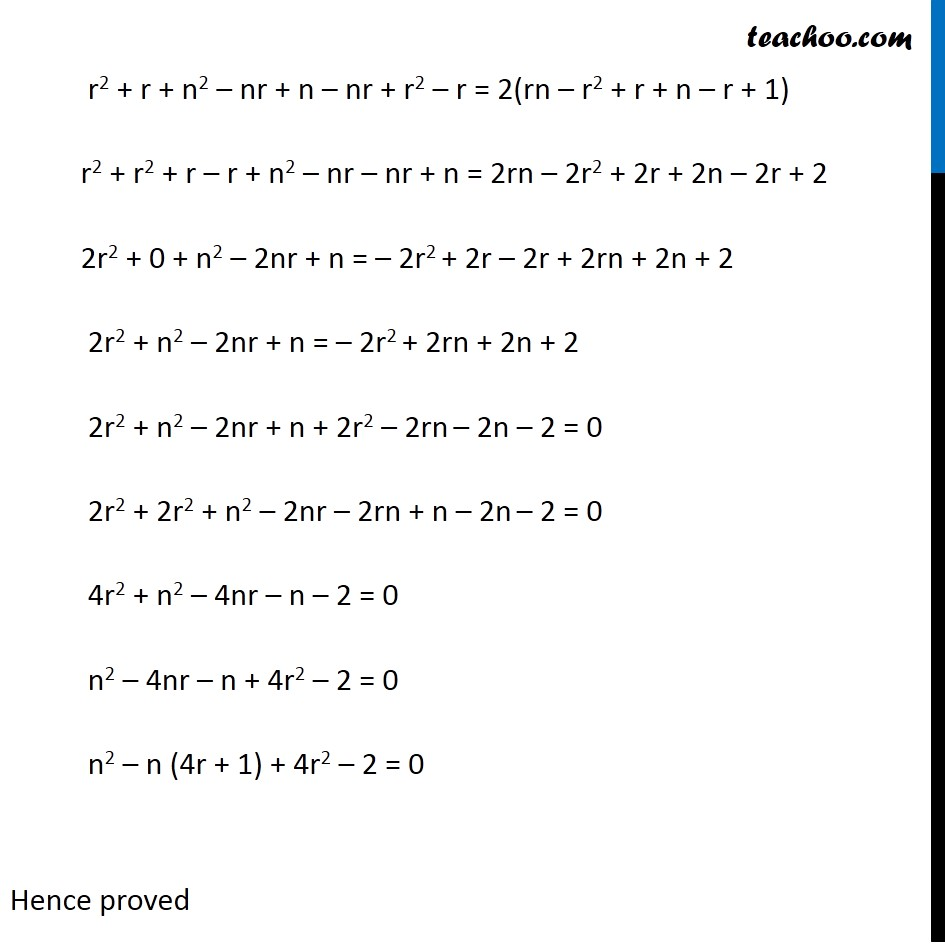1. Chapter 8 Class 11 Binomial Theorem (Deleted)
2. Serial order wise
3. Examples

Transcript

Example 11 If the coefficients of ar – 1, ar and ar + 1 in the expansion of (1 + a)n are in arithmetic progression, prove that n2 – n(4r + 1) + 4r2 – 2 = 0. We know that General term of (a + b)n Tr+1 = nCr an–r . br For (1 + a)n Putting a = 1 & b = a Tr + 1 = nCr (1)n – r . ar Tr + 1 = nCr ar Hence, Coefficient of ar = nCr Finding coefficient of ar – 1 Putting r = r – 1 in (1) T(r – 1) + 1 = nCr – 1 ar – 1 Tr = nCr – 1 ar – 1 ∴ Coefficient of ar – 1 = nCr – 1 For coefficient of ar + 1 Putting r = r + 1 in (1) T(r+1) + 1 = nCr + 1 ar + 1 Tr + 2 = nCr+1 ar + 1 ∴ Coefficient of ar + 1 = nCr + 1 Given that coefficient of ar–1 , ar , ar+1 are in AP i.e. nCr–1 , nCr , & nCr + 1 are in AP Therefore, common difference must be equal nCr – nCr – 1 = nCr+1 – nCr nCr + nCr = nCr+1 + nCr–1 2nCr = nCr+1 + nCr–1 nCr–1 + nCr+1 = 2nCr 𝑛!/(𝑟 − 1)![ 𝑛 − (𝑟 − 1)]! + 𝑛!/((𝑟 + 1)! (𝑛 − (𝑟 + 1))!) = 2 × 𝑛!/𝑟!(𝑛 − 𝑟)! n! (1/(𝑟−1)!(𝑛−𝑟+1)! " + " 1/((𝑟+1)!(𝑛−𝑟 −1)!)) = n! (2/𝑟!(𝑛 − 𝑟)!) 𝑛!/𝑛! (1/(𝑟−1)!(𝑛−𝑟+1)! " + " 1/((𝑟+1)!(𝑛−𝑟 −1)!)) = (2/𝑟!(𝑛−𝑟)!) 1/((r − 1)! (n − r + 1)(n − r)(n − r − 1)!) + 1/((r + 1)(r)(r − 1)! (n − r − 1)!) = 2/(r(r − 1)! (n − r)(n − r − 1)!) 1/(𝑟 − 1)!(𝑛 − 𝑟 − 1)! (1/((𝑛 − 𝑟 + 1)(𝑛 − 𝑟) ) "+" 1/((𝑟 + 1)(𝑟) )) = 2/((r − 1)! (n − r − 1) ! [𝑟(n − r)]) (𝑟−1)!(𝑛−𝑟+1)!/(𝑟−1)!(𝑛−𝑟+1)! (1/((𝑛 − 𝑟 + 1)(𝑛 − 𝑟) ) "+" 1/((𝑟 + 1)(𝑟) )) = 2/𝑟(n − r) 1/((𝑛 − 𝑟) (𝑛 − 𝑟 − 1) ) + 1/((𝑟 + 1) 𝑟) = 2/(𝑟 (𝑛 − 𝑟) ) ((𝑟 + 1)𝑟 + (𝑛 − 𝑟)(𝑛 − 𝑟 + 1))/( (𝑛 − 𝑟)(𝑛 − 𝑟 + 1) (𝑟 + 1)𝑟) = 2/( 𝑟(𝑛 − 𝑟) ) (r + 1) r + (n – r) (n – r + 1) = (2(n − r)(n − r + 1)(r + 1)(𝑟))/( 𝑟(n − r) ) (r + 1) r + (n – r) (n – r + 1)= 2(n – r + 1) (r + 1) r2 + r + n (n – r + 1) – r (n – r + 1) = 2 [r (n – r + 1) + 1(n – r + 1 )] r2 + r + n2 – nr + n – nr + r2 – r = 2(rn – r2 + r + n – r + 1) r2 + r2 + r – r + n2 – nr – nr + n = 2rn – 2r2 + 2r + 2n – 2r + 2 2r2 + 0 + n2 – 2nr + n = – 2r2 + 2r – 2r + 2rn + 2n + 2 2r2 + n2 – 2nr + n = – 2r2 + 2rn + 2n + 2 2r2 + n2 – 2nr + n + 2r2 – 2rn – 2n – 2 = 0 2r2 + 2r2 + n2 – 2nr – 2rn + n – 2n – 2 = 0 4r2 + n2 – 4nr – n – 2 = 0 n2 – 4nr – n + 4r2 – 2 = 0 n2 – n (4r + 1) + 4r2 – 2 = 0 Hence proved

Examples

Chapter 8 Class 11 Binomial Theorem (Deleted)
Serial order wise